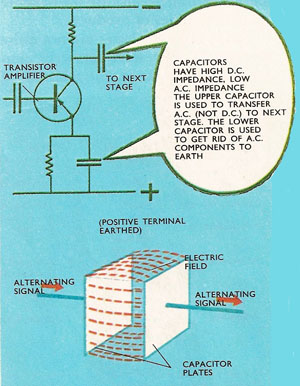A# impedanceIn a capacitor, current energy is temporarily stored as electric field energy.

Impedance (Z) is the quantity that measures the opposition of a circuit to the passage of an electric current and therefore determines the amplitude of the current. In a DC (direct current) circuit this is the resistance (R) alone. In an AC (alternating current) circuit, however, the reactance (X) also has to be taken into account, according to the equation Z 2 = R 2 + X 2, where Z is the impedance. The complex impedance is given by Z = R + iX, where i = √-1.

The real part of the complex impedance, the resistance, represents the loss of power according to Joule's law. The ratio of the imaginary part, the reactance, to the real part is an indication of the difference in phase between the voltage and the current.

## Forms of impedance

All types of opposition offered to electric currents in circuits are called impedance. Electrical resistors are only one of three kinds of impedances. The others are called inductors (coils of wire) or capacitors (plates separated by a thin insulating gap). Of these, only resistance can be a wasteful form of impedance. Electrons give up some of their energy to fixed atoms in the resistance wire. The electrons can never recover this energy, which is radiated away from the wire as heat. This is not wasteful if the heat is wanted – in an electric stove or electric fire for example, where the heating elements are electrical resistances.

In the two other forms of impedance the electric current has to give up some of its energy to overcome the impedance, but this energy is kept in a form which can be given back directly to the electric current. In a capacitor, the energy is stored in an electric field, and in an inductor, in a magnetic field. When these fields collapse, their energy can be given back to the electric current.

Pure resistance is the same whether the current is a steady one-way flow (direct current) or a to-and-fro flow (alternating current). But impedance of capacitors and inductors applies more to alternating currents than direct currents. The only impedance to a steady current offered by an inductance is its own resistance (no circuit component can be made completely free of resistance). All electric currents are surrounded by magnetic fields, but if the current is steady, the field is constant and requires no extra energy from the current to maintain it.

If the current is changing however, the field changes too. During part of a cycle of alternating currents the field will be built up to a maximum. Then the field starts to collapse to nothing as the current decreases to zero and starts flowing in the opposite direction. The field builds up and collapses in the opposite direction. Each time the field builds up the current gives some of its energy to the magnetic field. Each time the field collapses energy is given back to the current. This form of impedance, which is called inductive reactance, increases as the rate of change in current increases. It is proportional to the frequency of an alternating current.

Capacitors offer an infinitely large impedance to direct currents. They do not offer an infinitely large resistance, because this would imply that the capacitor was using up all the electrical energy supplied to it. Electrical charges accumulate on both sides of the capacitor, and an electric field appears in the space between the plates. A steady current cannot be transferred from one plate to another, so its impedance is said to be infinite to steady currents.

When the current changes, the charges on one side of the gap change. The field alters, and since the field acts as a link between the plates, it affects the charge on the plate. Electric charges flow to and from one plate as charges flow from and to the other plate. The higher the frequency of the current, the easier it is for variations on one side of the gap to be passed on to the other side. The impedance decreases as the frequency increases. The impedance of a capacitor is called capacitative resistance.

## Real and imaginary impedance

It is impossible to make inductors without resistance, or capacitors without a small amount of leakage resistance across the gap. Conversely, all resistance coils have a certain amount of inductance and capacitance. So the impedance of any electrical component is usually a complex mixture of all three kinds.

The real part of any impedance is the part which causes the irreversible change of electrical energy into heat energy. The other parts of the impedance are called imaginary because there is no real wastage of energy involved.